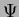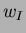Next: Introduction

# ANEM Interpolant for Fourth-Order PDEs

Internal Report

N. Sukumar
Theoretical and Applied Mechanics
Northwestern University
Evanston IL 60208

Date: December 4, 1997

A postscript version of this document.

### Abstract:

Farin  used the notion of Bézier simplices in Sibson coordinates  to propose ainterpolant. Theinterpolant has quadratic precision, and reduces to a cubic polynomial between adjacent nodes on the boundary. In this report, we present theformulation and propose a computational methodology for its implementation in the context of a Galerkin procedure for the solution of partial differential equations (PDEs). The approach involves the transformation of the original Bernstein basis functionsto new shape functions, such that the shape functions,,for nodeare directly associated with the three nodal degrees of freedom,,, respectively. Such a transformation renders the interpolant amenable to application in a Galerkin scheme for the solution of fourth-order elliptic PDEs. Numerical interpolation results have verified the smoothness and quadratic precision property of the interpolant, and currently its application to the biharmonic equation is in progress.Next: Introduction
N. Sukumar